Test: Equations- 2

# Test: Equations- 2

Test Description

## 40 Questions MCQ Test Business Mathematics and Logical Reasoning & Statistics | Test: Equations- 2

Test: Equations- 2 for CA Foundation 2023 is part of Business Mathematics and Logical Reasoning & Statistics preparation. The Test: Equations- 2 questions and answers have been prepared according to the CA Foundation exam syllabus.The Test: Equations- 2 MCQs are made for CA Foundation 2023 Exam. Find important definitions, questions, notes, meanings, examples, exercises, MCQs and online tests for Test: Equations- 2 below.
Solutions of Test: Equations- 2 questions in English are available as part of our Business Mathematics and Logical Reasoning & Statistics for CA Foundation & Test: Equations- 2 solutions in Hindi for Business Mathematics and Logical Reasoning & Statistics course. Download more important topics, notes, lectures and mock test series for CA Foundation Exam by signing up for free. Attempt Test: Equations- 2 | 40 questions in 40 minutes | Mock test for CA Foundation preparation | Free important questions MCQ to study Business Mathematics and Logical Reasoning & Statistics for CA Foundation Exam | Download free PDF with solutions
 1 Crore+ students have signed up on EduRev. Have you?
Test: Equations- 2 - Question 1

### 3x–4y+70z = 0,  2x+3y–10z = 0,  x+2y+3z = 13

Test: Equations- 2 - Question 2

### Monthly incomes of two persons are in the ratio 4 : 5 and their monthly expenses are in the ratio 7 : 9. If each saves Rs. 50 per month find their monthly incomes.

Test: Equations- 2 - Question 3

### Find the fraction which is equal to 1/2 when both its numerator and denominator are increased by 2. It is equal to 3/4 when both are incresed by 12.

Test: Equations- 2 - Question 4

The age of a person is twice the sum of the ages of his two sons and five years ago his age was thrice the sum of their ages. Find his present age.

Detailed Solution for Test: Equations- 2 - Question 4

Let the age of father be x and that of his son be a,b

We have x=2(a+b) and we have been given that 5 years ago we had the following situation which is given by the equation x-5=3(a+b-10)

On solving the above equations we obtain

a+b=25 thus the age of father is 50

Test: Equations- 2 - Question 5

A number between 10 and 100 is five times the sum of its digits. If 9 be added to it the digits are reversed find the number.

Test: Equations- 2 - Question 6

The wages of 8 men and 6 boys amount to Rs. 33. If 4 men earn Rs. 4.50 more than 5 boys determine the wages of each man and boy.

Test: Equations- 2 - Question 7

A number consisting of two digits is four times the sum of its digits and if 27 be added to it the digits are reversed. The number is :

Test: Equations- 2 - Question 8

Of two numbers, 1/5th of the greater is equal to 1/3rd of the smaller and their sum is 16.The numbers are:

Test: Equations- 2 - Question 9

Y is older than x by 7 years 15 years back X’s age was 3/4 of Y’s age. Their present ages are:

Test: Equations- 2 - Question 10

The sum of the digits in a three digit number is 12. if the digits are reversedthe number is increased by 495 but reversing only of ten’s and the unit digits increases the number by 36

Test: Equations- 2 - Question 11

Two numbers are such that twice the greater number exceeds twice the smaller one by 18 and 1/3 of the smaller and 1/5 of the greater number are together 21. The numbers are :

Test: Equations- 2 - Question 12

The demand and supply equations for a certain commodity are 4q + 7p = 17 and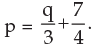respectively where p is the market price and q is the quantity then the equilibrium price and quantity are:

Test: Equations- 2 - Question 13

If the roots of the equation 2x2 + 8x – m3 = 0 are equal then value of m is

Test: Equations- 2 - Question 14

If 22x + 3 – 32. 2x + 1 = 0 then values of x are

Test: Equations- 2 - Question 15

The cost of 10 kg of apples is equal to the cost of 24 kg of rice. The cost of 6 kg of flour equals the cost of 2 kg of rice. The cost of each kg of flour is Rs.20.50. Find the total cost of 4 kg of apples, 3 kg of rice and 5 kg of flour?

Detailed Solution for Test: Equations- 2 - Question 15

Let the costs of each kg of apples and each kg of rice be Rs.a and Rs.r respectively.
10a = 24r and 6 * 20.50 = 2r
a = 12/5 r and r = 61.5
a = 147.6
Required total cost = 4 * 147.6 + 3 * 61.5 + 5 * 20.5
= 590.4 + 184.5 + 102.5 = Rs.877.40

Test: Equations- 2 - Question 16

If œ ß be the roots of the equation 2x2 – 4x – 3 = 0 the value of α2 + β2 is

Test: Equations- 2 - Question 17

If the sum of the roots of the quadratic equation ax2 + bx + c = 0 is equal to the sum of the squares of their reciprocals then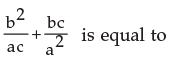Test: Equations- 2 - Question 18

The equation x2 –(p+4)x + 2p + 5 = 0 has equal roots the values of p will be.

Test: Equations- 2 - Question 19

The roots of the equation x2 + (2p–1)x + p2 = 0 are real if.

Test: Equations- 2 - Question 20

If x = m is one of the solutions of the equation 2x2 + 5x – m = 0 the possible values of m are

Test: Equations- 2 - Question 21

If p and q are the roots of x2 + x + 1 = 0 then the values of p3 + q3 becomes

Detailed Solution for Test: Equations- 2 - Question 21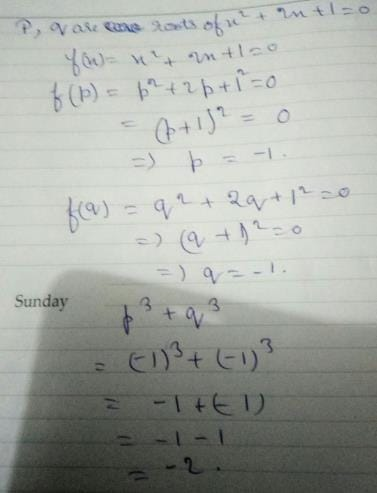Test: Equations- 2 - Question 22

If L + M + N = 0 and L M N are rationals the roots of the equation (M+N–L) x2 + (N+L–M)x + (L+M–N) = 0 are

Test: Equations- 2 - Question 23

If ∝ and ß are the roots of x2 = x+1 then value of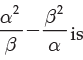Test: Equations- 2 - Question 24

If p ≠ q and p2 = 5p – 3 and q2 = 5q – 3 the equation having roots as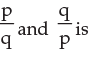Test: Equations- 2 - Question 25

If one rot of 5x2 + 13x + p = 0 be reciprocal of the other then the value of p is

Test: Equations- 2 - Question 26

A solution of the quadratic equation (a+b–2c)x2 + (2a–b–c)x + (c+a–2b) = 0 is

Test: Equations- 2 - Question 27

If the root of the equation x2–8x+m = 0 exceeds the other by 4 then the value of m is

Test: Equations- 2 - Question 28

The values of x in the equation 7(x+2p)2 + 5p2 = 35xp + 117p2 are

Test: Equations- 2 - Question 29

The solutions of the equation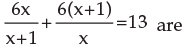Test: Equations- 2 - Question 30

The satisfying values of x for the equation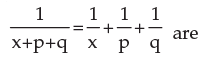Test: Equations- 2 - Question 31

Time taken by a train to slow down from 80 kmh-1 to 20 kmh-1 with a uniform deceleration of 2 ms-2 is

Test: Equations- 2 - Question 32

The values of x satisfying the equation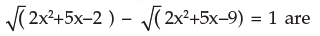Test: Equations- 2 - Question 33

The solution of the equation 3x2–17x + 24 = 0 are

Test: Equations- 2 - Question 34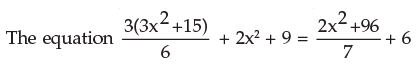has got the solution as

Test: Equations- 2 - Question 35

The equation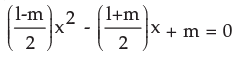has got two values of x to satisfy the equation given as

Test: Equations- 2 - Question 36

Te sum of two numbers is 8 and the sum of their squares is 34. Taking one number as x form an equation in x and hence find the numbers. The numbers are

Test: Equations- 2 - Question 37

The difference of two positive integers is 3 and the sum of their squares is 89. Taking the smaller integer as x form a quadratic equation and solve it to find the integers. The integers are.

Test: Equations- 2 - Question 38

Five times of a positive whole number is 3 less than twice the square of the number. The number is

Test: Equations- 2 - Question 39

The area of a rectangular field is 2000 sq.m and its perimeter is 180m. Form a quadratic equation by taking the length of the field as x and solve it to find the length and breadth of the field. The length and breadth are

Detailed Solution for Test: Equations- 2 - Question 39

Let the length be l and the breadth be b. now area = 2000 sq.m so length * breadth = 2000; l*b=2000.

now perimeter = 180m

so 2 ( l + b ) =180;

l + b = 90;

l = 90 - b.

substituting this value of l in l * b = 2000,

( 90 - b ) * b = 2000;

90b - b^2 = 2000;

b^2 - 90b + 2000 = 0;

b^2 - 40b - 50b - 2000 = 0;

b ( b - 40 ) - 50( b - 40 ) = 0;

( b - 40 )( b - 50 ) = 0;

b = 40 or b = 50;

so l = 40 or l = 50 but the longer side is considered the length and the shorter one the breadth.

so length = 50 m and breadth = 40 m

Test: Equations- 2 - Question 40

Two squares have sides p cm and (p + 5) cms. The sum of their squares is 625 sq. cm. The sides of the squares are

## Business Mathematics and Logical Reasoning & Statistics

130 videos|150 docs|88 tests
Information about Test: Equations- 2 Page
In this test you can find the Exam questions for Test: Equations- 2 solved & explained in the simplest way possible. Besides giving Questions and answers for Test: Equations- 2, EduRev gives you an ample number of Online tests for practice

## Business Mathematics and Logical Reasoning & Statistics

130 videos|150 docs|88 tests(Scan QR code)